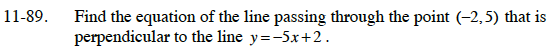Home > CCA2 > Chapter 11 > Lesson 11.3.1 > Problem11-89

11-89.

Find the equation of the line passing through the point (−2, 5) that is perpendicular to the line y = −5x + 2. Homework Help ✎A perpendicular line has a slope that is the opposite reciprocal of the given line.

Using y = mx + b, substitute the m, y, and x and solve for b.

$y= \frac{ 1 }{ 5} x+ \frac{ 27 }{ 5}$